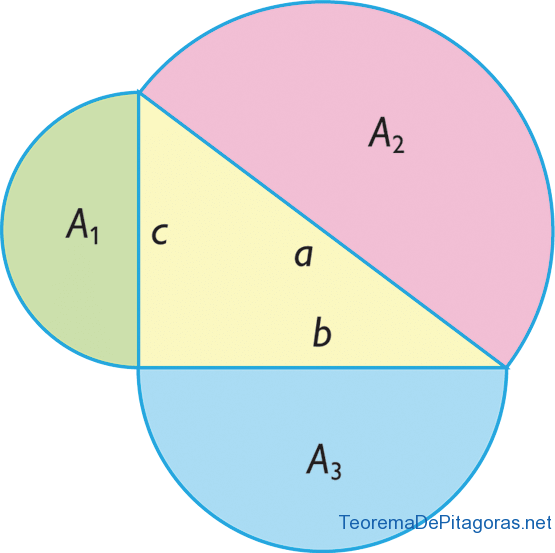# Pythagorean Theorem Proofs

The most well-known and widely used proof of the Pythagorean Theorem is the one which relates the areas of the squares that have, as their sides, the two legs and the hypotenuse of the triangle. We have referenced this proof in an older post where we have also provided a video that explains this proof of the Pythagorean Theorem in a simple way.However, what we will discuss in this article is the different proofs that have been done on the Pythagorean Theorem, since many mathematicians have demonstrated this famous theorem in different ways. Let’s look at some of the most well-known proofs of the Pythagorean Theorem without going into detail; in later posts, we’ll look at each one specifically and explain them in full detail.

• Pythagoras’ own proof
• Euclid’s proof
• Pappus’ proof